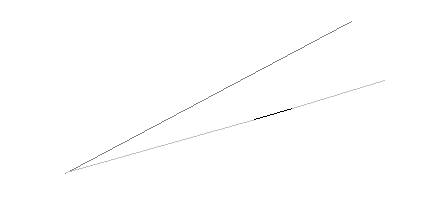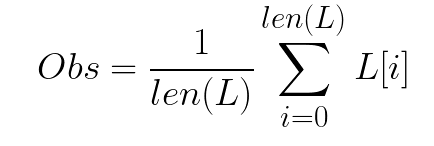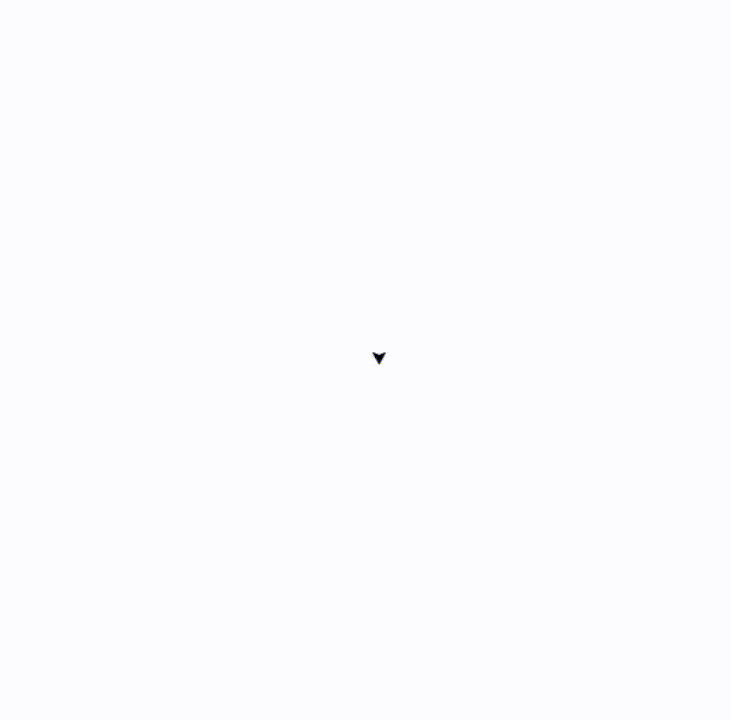# Image Processing in Python — Draw Aesthetic Portraits Using Only Nails and a Thread | by Ilias Nahmed | Aug, 2022

## Unleash the artist that lives within you.

Artists have become more and more creative, so much so that they might have us thinking: “how did they even know they could do that”. One way of drawing portraits that have become pretty famous on Instagram reels and Tiktok is doing it with a long thread, and a set of nails. The artist hammers the nails all over the circumference of a circular canvas, then starts linking the nails with the thread. While doing so, the image of the person drawn starts coming into being.

Having no talent whatsoever in drawing, I thought that, given the order in which the nails should be linked, even I could draw aesthetic portraits. All I had to do was to write a code that would provide me with this order of nails.

The main idea is quite a naive one. We take a grayscale picture and hammer imaginary nails into the circumference of the inscribed circle. Then, starting from a random nail, we calculate the obscurity of the line between this nail and all the other nails. The most obscure line will then be drawn in our canvas. We repeat this step hundreds of times, then we will acquire our final image.

## What is an image to the computer’s eyes?

To implement this idea, we must imagine that the image is a matrix in which the elements are the pixels. Each pixel is coded in a 3-elements array : its RGB code. It is a code that denotes the intensity of the 3 primary colors: red, green, and blue.

In fact, since every color is just a combination of these three colors, we can define images by this type of arrays. We should note that the intensity of each color is an int value between 0 and 255, with 0 being the lowest intensity, and 255 the highest.

The line linking every two nails can be seen as a vector of pixels. Evaluating its obscurity is the same as calculating the norm of the vector. The usual norms we might think of are the Euclidian norm, the L1-norm, and the infinite norm.

However, the infinite norm is not a precise one, as it will make us draw every line where there is a black pixel, even if it is the only non-white pixel on the line.The obscurity of the upper line is inferior to the bottom line’s if we use the infinite norm, however, the upper line is overall darker than the other, hence it is the one that needs to be drawn.

On the other hand, the Euclidian norm would be quite costly, as we are looking to repeat this process a few hundreds of times. That is why we will go with the L1-norm: we will be adding the value of the intensities of each pixel in the imaginary line, then in order to normalize, we will be dividing by the number of the pixels in the line (because There will be lines longer, yet not as obscure as other ones).

The line with the lowest “norm” is the most obscure one.Formula of obscurity, L being the line vector.

In each step, after selecting a line from the image, we will be turning the color of that very same line into white, so that we will not be drawing the same line again and again.

We will be using 3 main libraries: Numpy, Sci-kit Image (skimage), and Turtle.

Skimage will allow us to process the image. It reads the picture (a matrix of RGB codes). It can also provide us with a vector of the coordinates of all pixels that are linking two pixels, which will be really useful for us in order to calculate the obscurity of the lines.

Numpy will allow us to do some maths operations, such as calculating the norm of the vector (obscurity of the lines) and determining the coordinates of the nails using angles and “cos” and “sine” functions.

Finally, Turtle will allow us to draw the final image. What is interesting with Turtle is that it shows the process of the drawing, so we do not have to wait for the code to finish in order to see the result.

We will first import the three previous libraries. Then, we will read the image we will be drawing and crop it into a square. Next, we will hammer our imaginary nails. We will be using 300 nails.

We open the canvas we will be drawing on with Turtle. However, this library uses coordinates as a cartesian grid: the 0 is in the center, the x-axis goes from left to right, and the y axis from bottom to top. But since the image we are working on is a matrix in a way, then the 0 is in the top left corner and the y axis is towards the bottom. We will need to translate our screen then.

In order to get the order of nails we will be linking, we will be concatenating the nails’ numbers in order into a string.

Now let’s get to the main part: the for loop.

We will start from the first nail in our list, which we will call `next_nail`. The first for loop allows us to choose the number of iterations we will be doing. It is basically the number of links we will be drawing. The nested for loop allows us to search through all the other nails which one is linked to our `next_nail` with the most obscure line. Hence, we will be comparing the obscurity parameter. `rr1`,`cc1` is the vector of pixels we got with the `draw.line` function of the `skimage` library.

Afterward, we calculate the obscurity: it is the A/B parameter. Since we are looking for the most obscure line, we need the smallest A/B value, hence, in the j-loop, we update each time parameter `next_nail` with the best nail we can find, and the `best_line` with the line between our nail and `next_nail`. Finally, we draw in our Turtle canvas the `best_line`.

Note that we are using the “`graph_nails`“ coordinates for the reason we specified upwards.The making of Lily Collins’ portrait